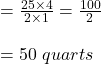## Mrs. Lopez made 12 ½ gallons of sweet tea for a celebration. How many quarts of sweet tea did Mrs. Lopez make?

Question

Mrs. Lopez made 12 ½ gallons of sweet tea for a celebration. How many quarts of sweet tea did Mrs. Lopez make?

in progress 0
6 months 2021-08-09T16:29:57+00:00 1 Answers 7 views 0

Mrs. Lopez made 50 quarts of sweet tea

Step-by-step explanation:

A gallon and a quart are two of the five US standard volumes of measurement. In relation to litres, a quart is 946.352946 millilitres while a gallon is  3.7854118 litres.

The relationship between The gallon and The quarts is given as 4:1

This means that for every 4  quarts, there is 1 gallon.

Now, let us find the number of quarts in 12¹/₂ gallons as follows:

1 gallon = 4 quarts

∴ 12¹/₂ gallons = 12¹/₂ × 4 quarts

converting the mixed fraction to improper fraction and multiplying:

= ²⁵/₂ × ⁴/₁